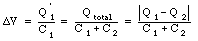## Solutions Physics 122 Midterm Exam # 2

Problem 1

Due to the symmetry of the arrangement, the potential difference across C' must be equal to zero. The circuit shown is thus equivalent to two parallel circuits of two capacitors in series each. The total capacitance of two capacitors of capacitance C in series is equal to: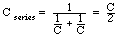By connecting two of these capacitors in parallel we obtain a circuit with a total capacitance equal to the sum of the individual capacitances: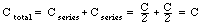Problem 2

The voltage across R2 is equal to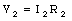This is also the voltage across resistor R1 and R3. The current through resistor R1 is thus equal toThe current through resistor R3 is thus equal toThe current through resistor R4 is equal to the sum of the current through the other resistors. Thus: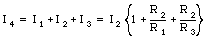The voltage across resistor R4 is thus equal to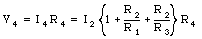The total voltage across the external terminals is thus equal to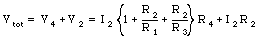Problem 3

The capacitor is initially uncharged. The potential difference across it is thus equal to 0 V. At the moment the switch is closed, the entire emf will be across the resistors. The current though R1 will thus be equal to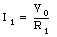Problem 4

Consider the branch method to solve this problem. The branch method immediately gives us the following relation between the three currents:Now consider the left loop. Applying Kirchoff's second rule we obtain: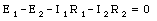For the right loop we obtain: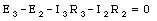From these three relations we can conclude that: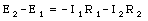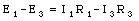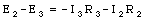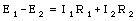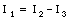Problem 5

Consider the following statements:

• The current density is defined as the current per unit area. Since the current is a vector (it has a direction), the current density is also a vector.
• For a superconductor the resistivity is equal to zero. Thus, the temperature coefficient, defined as the changed in the resistivity per unit temperature, is equal to zero.
• The resistance of a wire is proportional to its length. If you reduce the length by a factor of two, the resistance will also be reduced by a factor of two. However, the resistivity is not a function of the dimensions; it is only a function of the material and the temperature of the material. It is thus not effected by the change in the dimensions.
• The resistivity of conductors is typically in the order of 10^-8 [Omega]m.
• The average time interval between collisions is nearly independent of the applied voltage.
• The typical drift velocity of an electron in a conductor is about 10^-2 m/s.

Problem 6

The total resistance of each wire in the extension cord is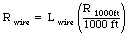where Lwire is the length of the wire in feet. The total current drawn by the appliances is equal to the sum of the currents: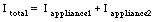The power lost by the current when is passes through each of the wires of the extension cord is equal to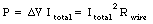Since there are two wires in the extension cord, the total power lost is twice this:Problem 7

NOTE: the following solution is correct for the circuit shown on the assignment of Frank Wolfs. Different diagrams might appear on different assignments, but the approach will be the same.

The net current at each branch must be zero. This immediately implies that: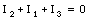or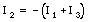Now consider the loop with resistors 1 and 2 (and go around this loop in a direction given by the current I2). Kirchhoffs' law states that the net emf around this loop must be equal to zero. Thus: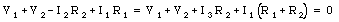Do the same thing for the loop with resistors 2 and 3 (and go around this loop in a direction given by the current I2):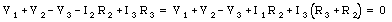We now have two equations with two unknown. The current I1 is equal toProblem 8

In the final configuration, the voltage across each capacitor will be the same. The magnitude of the total charge on the top and on the bottom plates after the connection is made is equal to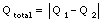The final charges on capacitor 1 and 2 are Q1' and Q2'. Since the potential difference across each capacitor must be the same, we require that: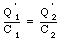At the same time we also require that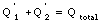These last two equations can be used to solve for Q1' and Q2':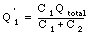and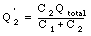The potential difference across the capacitors is thus equal to